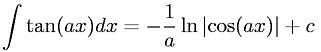Equations > Calculus > Integration/Integrals > Integral of tangent

### Integral of tangentLatex Code:

MathML Code:

 $\int \mathrm{tan}\left(\mathrm{ax}\right)\mathrm{dx}=-\frac1a\mathrm{ln}|\mathrm{cos}\left(\mathrm{ax}\right)|+c$

MathType 5.0: